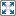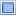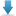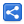# TypeScript - Function Types

[Updated: Oct 6, 2018, Created: Sep 20, 2018]

## Function parameters and return types

As we have seen in previous tutorials, TypeScript allows us to specify types with the function parameters and for return values. For example:

```function getMessage(count: number): string{
return `Message no \${count}`;
}
```

## Reusable Function type

We also have seen that interfaces can be used to describe reuseable type of a function.

Following example shows how to assign an interface type to an anonymous function (created via function expression)

```interface MsgFunc {
(count: number): string;
}

let f: MsgFunc = function (count: number): string {
return `Message no \${count}`;
}
let s: string = f(1);
console.log(s);
```

#### Output

`Message no 1`

## Directly specifying the Function type

TypeScript also allows to assign the type to a function without creating an interface. For example

```let f: (ct: number) => string = function (count: number): string {
return `Message no \${count}`;
}
let s: string = f(1);
console.log(s);
```

#### Output

`Message no 1`

Considering the part (count: number) => string, the left side specifies the parameter types and the right side of the arrow (=>) specifies the return type.

We can also specify direct function type as a function parameter:

```function process(f: (ct: number) => string) {
let s: string = f(1);
console.log(s);
}

process(function (count: number): string {
return `Message no \${count}`;
});
```

#### Output

`Message no 1`

## Without Function type

If we don't use any function types and just use parameter/return types then TypeScript can still infer them, for example:

```let square = function (x: number): number {
return x * x;
}
let num: number = 3;
square(num);

```

If we try to use wrong type TypeScript will warn us:

```let square = function (x: number): number {
return x * x;
}
square("hi")
```

#### Output

`function-type-inference2.ts(4,8): error TS2345: Argument of type '"hi"' is not assignable to parameter of type 'number'.`

## Function types in other places

Function types can appear in other places too. Following example shows how to use function type as a class member:

```class Util {
x: number;
process: (ct: number) => string;
}

let u: Util = new Util();
u.x = 3;
u.process = function (n: number): string {
return `Message no \${n}`
};

console.log(u);
console.log(u.process(4));```

#### Output

`Util { x: 3, process: [Function] }Message no 4`

## Example Project

Dependencies and Technologies Used:

• TypeScript 3.0.3
 Function TypesSelect AllDownload• typescript-function-type
• direct-function-types.ts

 Share